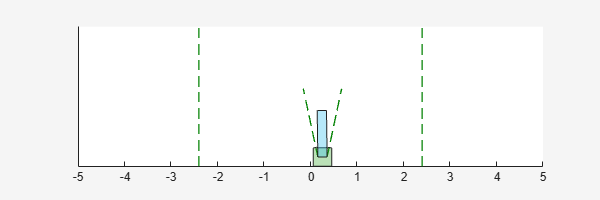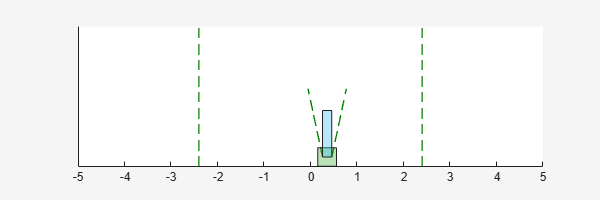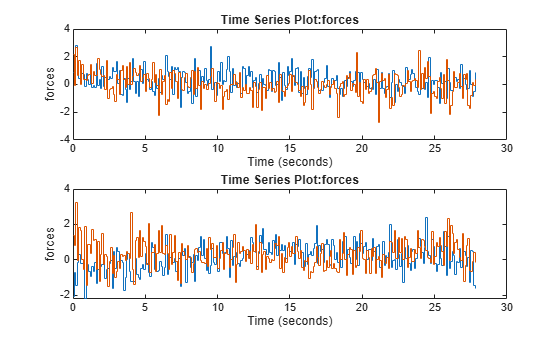# sim

Simulate trained reinforcement learning agents within specified environment

## Syntax

``experience = sim(env,agents)``
``experience = sim(agents,env)``
``env = sim(___,simOpts)``

## Description

example

````experience = sim(env,agents)` simulates one or more reinforcement learning agents within an environment, using default simulation options.```
````experience = sim(agents,env)` performs the same simulation as the previous syntax.```
````env = sim(___,simOpts)` uses the simulation options object `simOpts`. Use simulation options to specify parameters such as the number of steps per simulation or the number of simulations to run. Use this syntax after any of the input arguments in the previous syntaxes.```

## Examples

collapse all

Simulate a reinforcement learning environment with an agent configured for that environment. For this example, load an environment and agent that are already configured. The environment is a discrete cart-pole environment created with `rlPredefinedEnv`. The agent is a policy gradient (`rlPGAgent`) agent. For more information about the environment and agent used in this example, see Train PG Agent to Balance Cart-Pole System.

```rng(0) % for reproducibility load RLSimExample.mat env```
```env = CartPoleDiscreteAction with properties: Gravity: 9.8000 MassCart: 1 MassPole: 0.1000 Length: 0.5000 MaxForce: 10 Ts: 0.0200 ThetaThresholdRadians: 0.2094 XThreshold: 2.4000 RewardForNotFalling: 1 PenaltyForFalling: -5 State: [4×1 double] ```
`agent`
```agent = rlPGAgent with properties: AgentOptions: [1×1 rl.option.rlPGAgentOptions] UseExplorationPolicy: 1 ObservationInfo: [1×1 rl.util.rlNumericSpec] ActionInfo: [1×1 rl.util.rlFiniteSetSpec] SampleTime: 0.1000 ```

Typically, you train the agent using `train` and simulate the environment to test the performance of the trained agent. For this example, simulate the environment using the agent you loaded. Configure simulation options, specifying that the simulation run for 100 steps.

`simOpts = rlSimulationOptions('MaxSteps',100);`

For the predefined cart-pole environment used in this example. you can use `plot` to generate a visualization of the cart-pole system. When you simulate the environment, this plot updates automatically so that you can watch the system evolve during the simulation.

`plot(env)`Simulate the environment.

`experience = sim(env,agent,simOpts)````experience = struct with fields: Observation: [1×1 struct] Action: [1×1 struct] Reward: [1×1 timeseries] IsDone: [1×1 timeseries] SimulationInfo: [1×1 struct] ```

The output structure `experience` records the observations collected from the environment, the action and reward, and other data collected during the simulation. Each field contains a `timeseries` object or a structure of `timeseries` data objects. For instance, `experience.Action` is a `timeseries` containing the action imposed on the cart-pole system by the agent at each step of the simulation.

`experience.Action`
```ans = struct with fields: CartPoleAction: [1×1 timeseries] ```

Simulate an environment created for the Simulink® model used in the example Train Multiple Agents to Perform Collaborative Task, using the agents trained in that example.

Load the agents in the MATLAB® workspace.

`load rlCollaborativeTaskAgents`

Create an environment for the `rlCollaborativeTask` Simulink® model, which has two agent blocks. Since the agents used by the two blocks (`agentA` and `agentB`) are already in the workspace, you do not need to pass their observation and action specifications to create the environment.

`env = rlSimulinkEnv('rlCollaborativeTask',["rlCollaborativeTask/Agent A","rlCollaborativeTask/Agent B"]);`

Load the parameters that are needed by the `rlCollaborativeTask` Simulink® model to run.

`rlCollaborativeTaskParams`

Simulate the agents against the environment, saving the experiences in `xpr`.

`xpr = sim(env,[agentA agentB]);`

Plot actions of both agents.

```subplot(2,1,1); plot(xpr(1).Action.forces) subplot(2,1,2); plot(xpr(2).Action.forces)```## Input Arguments

collapse all

Environment in which the agents act, specified as one of the following kinds of reinforcement learning environment object:

When `env` is a Simulink environment, calling `sim` compiles and simulates the model associated with the environment.

Agents to simulate, specified as a reinforcement learning agent object, such as `rlACAgent` or `rlDDPGAgent`, or as an array of such objects.

If `env` is a multi-agent environment created with `rlSimulinkEnv`, specify agents as an array. The order of the agents in the array must match the agent order used to create `env`. Multi-agent simulation is not supported for MATLAB environments.

For more information about how to create and configure agents for reinforcement learning, see Reinforcement Learning Agents.

Simulation options, specified as an `rlSimulationOptions` object. Use this argument to specify options such as:

• Number of steps per simulation

• Number of simulations to run

For details, see `rlSimulationOptions`.

## Output Arguments

collapse all

Simulation results, returned as a structure or structure array. The number of rows in the array is equal to the number of simulations specified by the `NumSimulations` option of `rlSimulationOptions`. The number of columns in the array is the number of agents. The fields of each `experience` structure are as follows.

Observations collected from the environment, returned as a structure with fields corresponding to the observations specified in the environment. Each field contains a `timeseries` of length N + 1, where N is the number of simulation steps.

To obtain the current observation and the next observation for a given simulation step, use code such as the following, assuming one of the fields of `Observation` is `obs1`.

```Obs = getSamples(experience.Observation.obs1,1:N); NextObs = getSamples(experience.Observation.obs1,2:N+1);```
These values can be useful if you are writing your own training algorithm using `sim` to generate experiences for training.

Actions computed by the agent, returned as a structure with fields corresponding to the action signals specified in the environment. Each field contains a `timeseries` of length N, where N is the number of simulation steps.

Reward at each step in the simulation, returned as a `timeseries` of length N, where N is the number of simulation steps.

Flag indicating termination of the episode, returned as a `timeseries` of a scalar logical signal. This flag is set at each step by the environment, according to conditions you specify for episode termination when you configure the environment. When the environment sets this flag to 1, simulation terminates.

Information collected during simulation, returned as one of the following:

• For MATLAB environments, a structure containing the field `SimulationError`. This structure contains any errors that occurred during simulation.

• For Simulink environments, a `Simulink.SimulationOutput` object containing simulation data. Recorded data includes any signals and states that the model is configured to log, simulation metadata, and any errors that occurred.

## Version History

Introduced in R2019a